A uniform bar of length 6a and mass 8m lies on a smooth horizontal table. Two-point
masses m and 2m moving in the same horizontal plane with speeds 2v and v
respectively strike the bar as shown below in the figure and stick to the bar after the collision. Denoting angular velocity about the center of mass, total energy, and center of mass velocity of $\omega$, E and ${\mathrm{v}}_{\mathrm{c}}$ respectively, we have after collision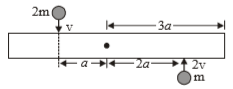1.

2.

3.

4.

Concept Questions :-

Angular momentum
High Yielding Test Series + Question Bank - NEET 2020

Difficulty Level:

A stone is projected from a horizontal plane. It attains maximum height 'H' & strikes a stationary smooth wall & falls on the ground vertically below the maximum height. Assume the collision to be elastic, the height of the point on the wall where ball will strike is: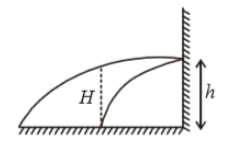(1) $\frac{H}{2}$

(2) $\frac{H}{4}$

(3) $\frac{3H}{4}$

(4) None of these

Concept Questions :-

Linear momentum
High Yielding Test Series + Question Bank - NEET 2020

Difficulty Level:

A train of mass M is moving on a circular track of radius 'R' with constant speed v. The length of the train is half of the perimeter of the track. The linear momentum of the train will be

1. 0

2. $\frac{2\mathrm{Mv}}{\mathrm{\pi }}$

3. MvR

4. Mv

Concept Questions :-

Linear momentum
High Yielding Test Series + Question Bank - NEET 2020

Difficulty Level:

A 2kg toy car can move along an x-axis. The graph shows force ${\mathrm{F}}_{\mathrm{x}}$, acting on the car which begins at rest at time t = 0. The velocity of the car at t = 10 s is :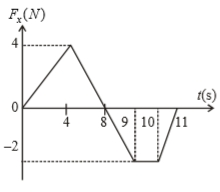1. $-\stackrel{^}{\mathrm{i}}$ m/s

2.  m/s

3.  m/s

4.  m/s

Concept Questions :-

Linear momentum
High Yielding Test Series + Question Bank - NEET 2020

Difficulty Level:

A particle of mass m is moving at speed v perpendicular to a rod of length d and mass M = 6m which pivots around a frictionless axle running through its centre. It strikes and sticks to the end of the rod. The moment of inertia of the rod about its centre is $M{d}^{2}/12$. Then the angular speed  of the system just after the collision is: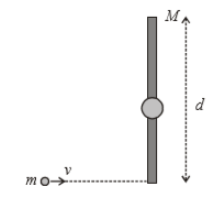1. 2v/3d

2. 2v/d

3. v/d

4. v

Concept Questions :-

Angular momentum
High Yielding Test Series + Question Bank - NEET 2020

Difficulty Level:

In the figure shown, a small solid spherical ball of mass 'm' can move without sliding in a fixed semicircular track of radius R in vertical plane. It is released from the top. The resultant force on the ball at the lowest point of the track is :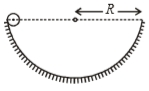1.

2.

3.

4. zero

Concept Questions :-

Rolling motion
High Yielding Test Series + Question Bank - NEET 2020

Difficulty Level:

A uniform thin rod is bent in the form of closed loop ABCDEFA as shown in the figure. The ratio of inertia of the loop about x-axis to that about y-axis is: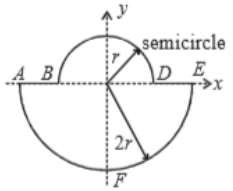1.  >1

2.  <1

3.  =1

4.  =1/2

Concept Questions :-

Moment of inertia
High Yielding Test Series + Question Bank - NEET 2020

Difficulty Level:

Four spheres of diameter 2a and mass M are placed with their centres on the four corners of a square of side b. Then the moment of inertia of the system about an axis about one of the sides of the square is

(1) $M{a}^{2}+2M{b}^{2}$

(2) $M{a}^{2}$

(3) $M{a}^{2}+4M{b}^{2}$

(4) $\frac{8}{5}M{a}^{2}+2M{b}^{2}$

Concept Questions :-

Moment of inertia
High Yielding Test Series + Question Bank - NEET 2020

Difficulty Level:

A body of mass (m) elastically collides with another stationary body of mass 3m. The fractional loss of K.E. of the body is:

1.  $\frac{8}{9}$

2.  $\frac{3}{4}$

3.  $\frac{3}{5}$

4.  $\frac{4}{7}$

Concept Questions :-

Linear momentum
High Yielding Test Series + Question Bank - NEET 2020

Difficulty Level:

A hollow sphere is rolling without slipping on the inclined plane. The minimum coefficient of friction is :

1. $\frac{2}{5}\mathrm{tan}\theta$

2. $\frac{2}{3}\mathrm{tan}\theta$

3. $\frac{3}{4}\mathrm{tan}\theta$

4. $\frac{5}{7}\mathrm{tan}\theta$

Concept Questions :-

Rolling motion